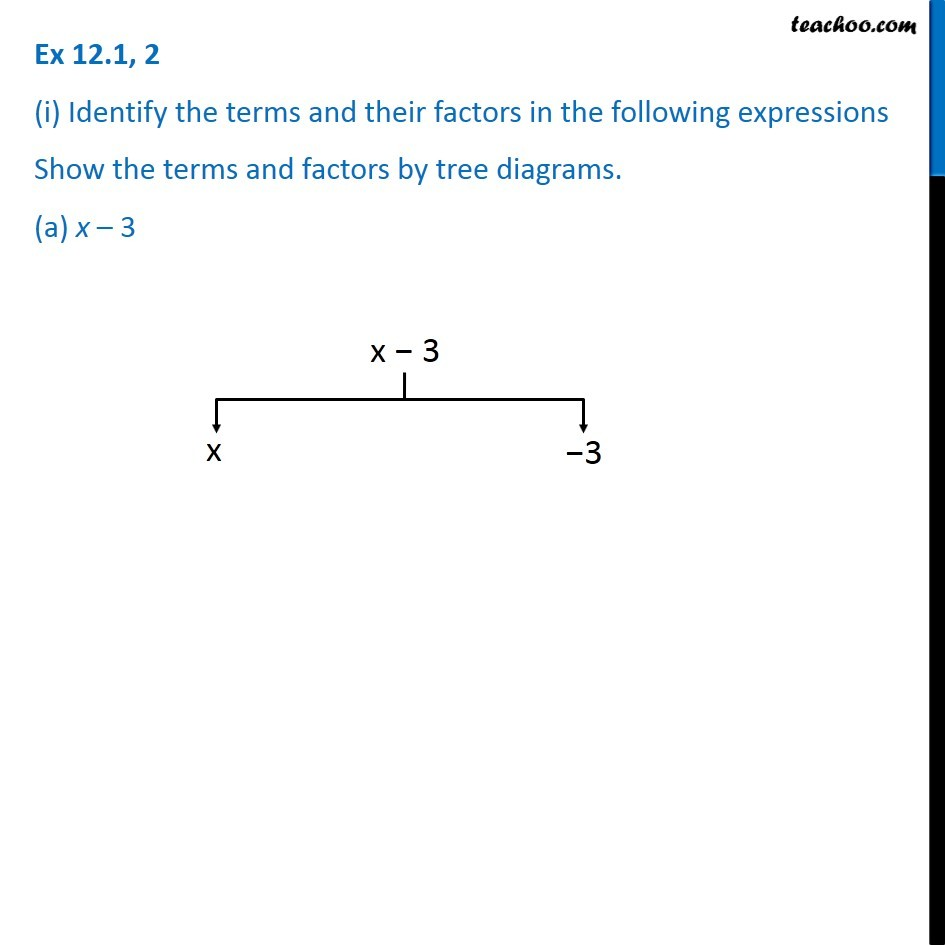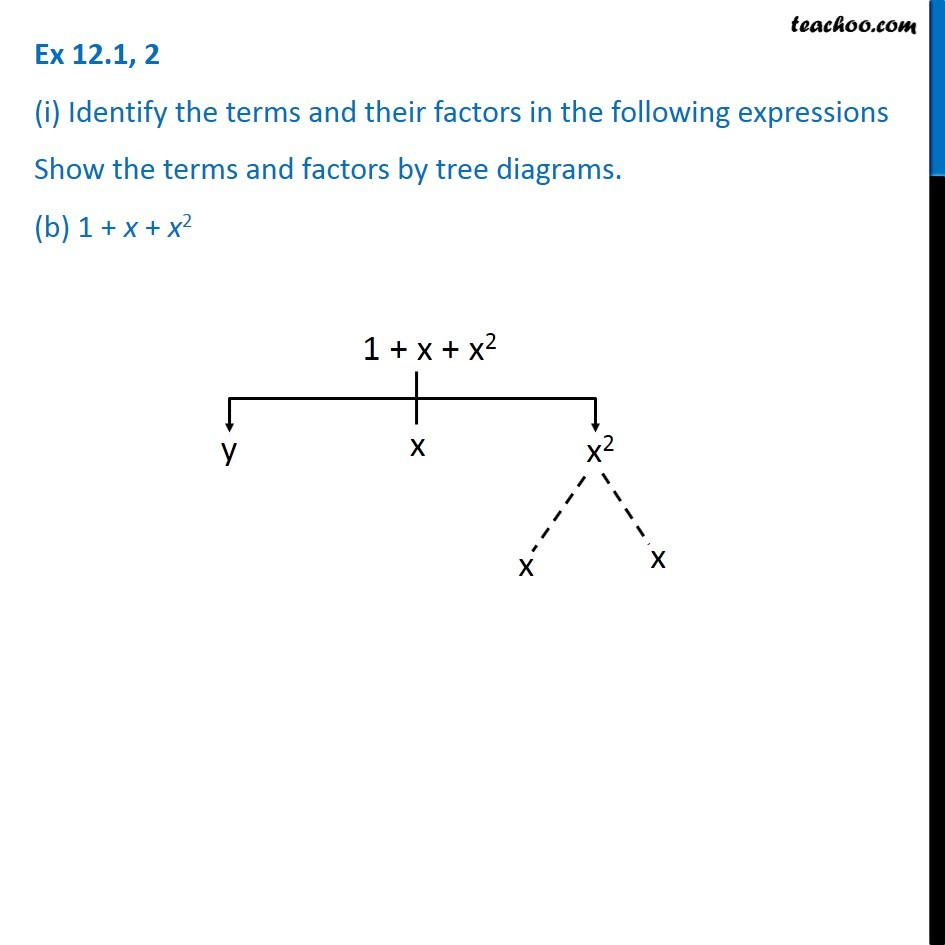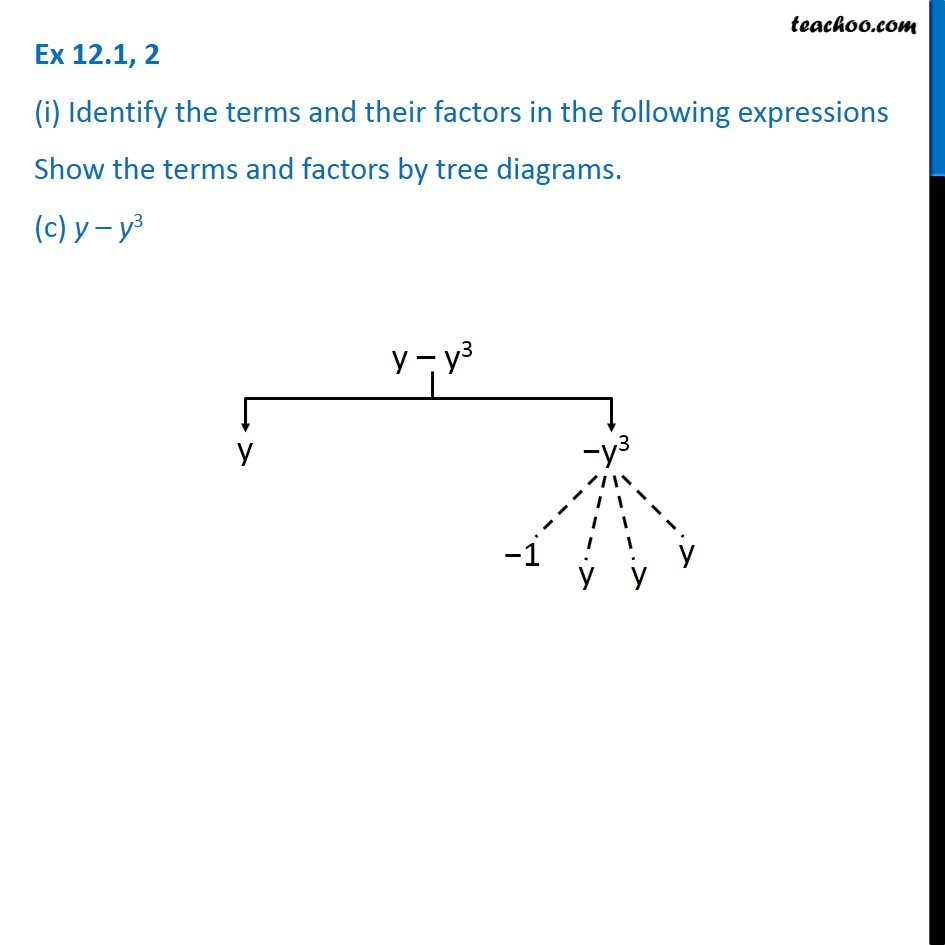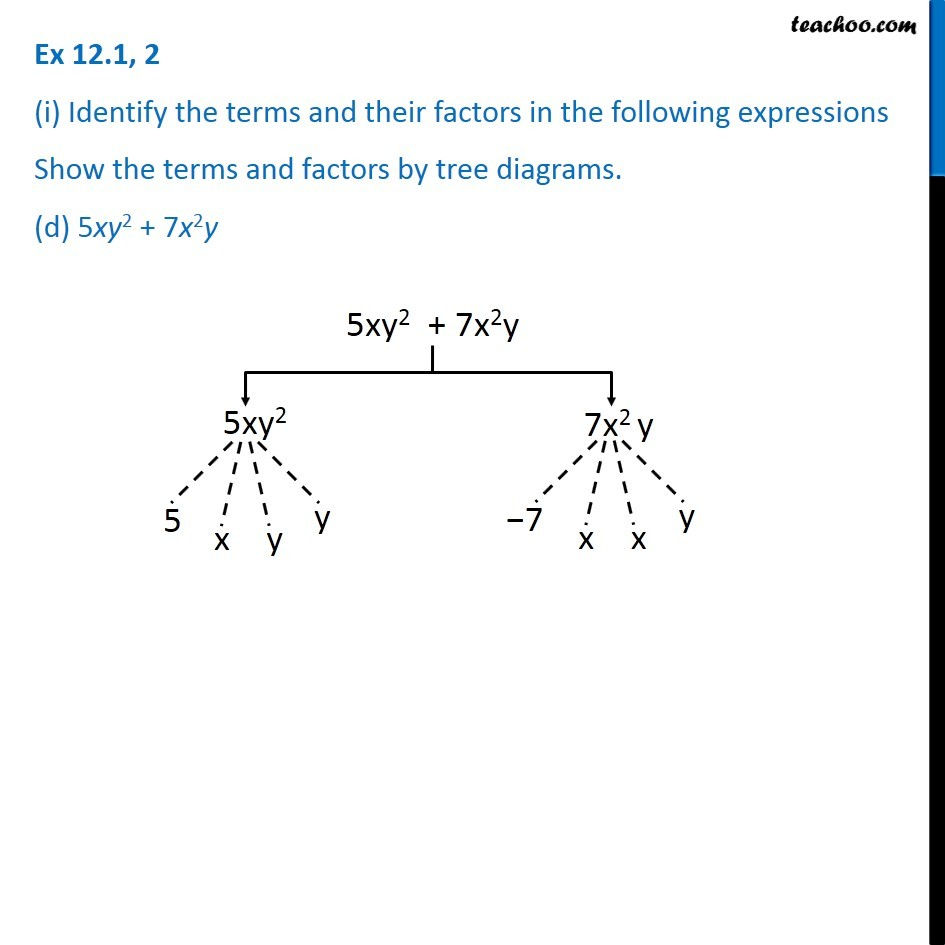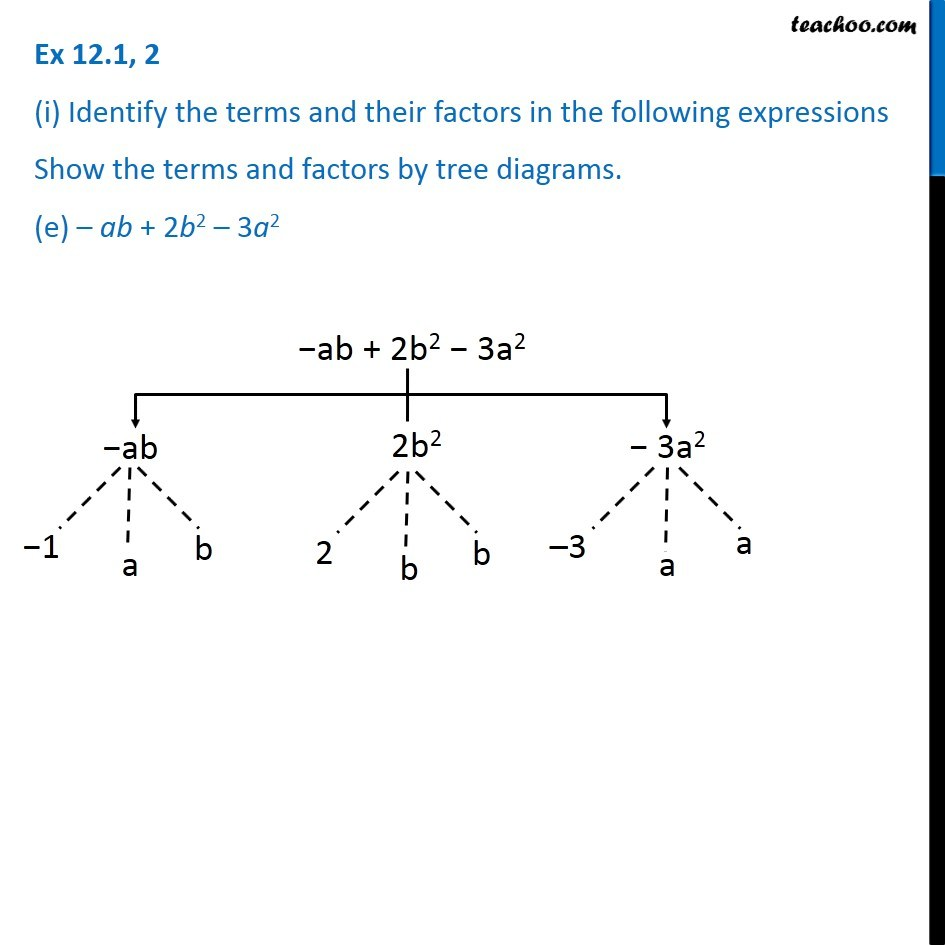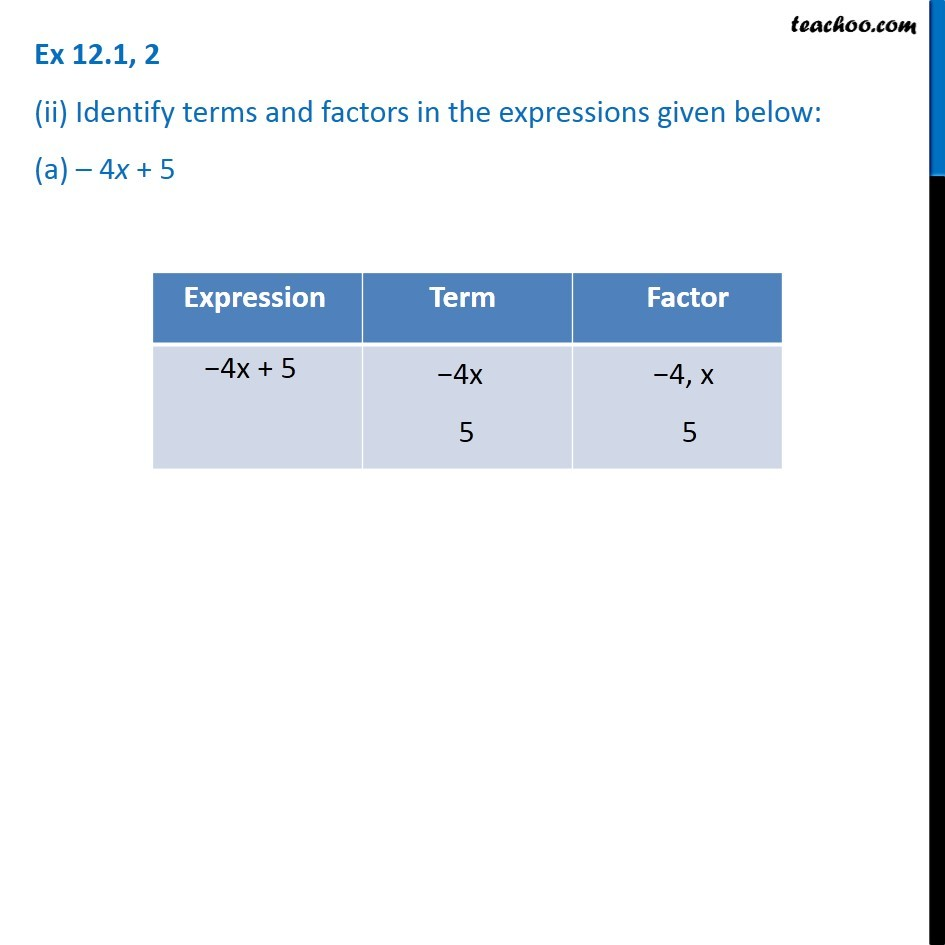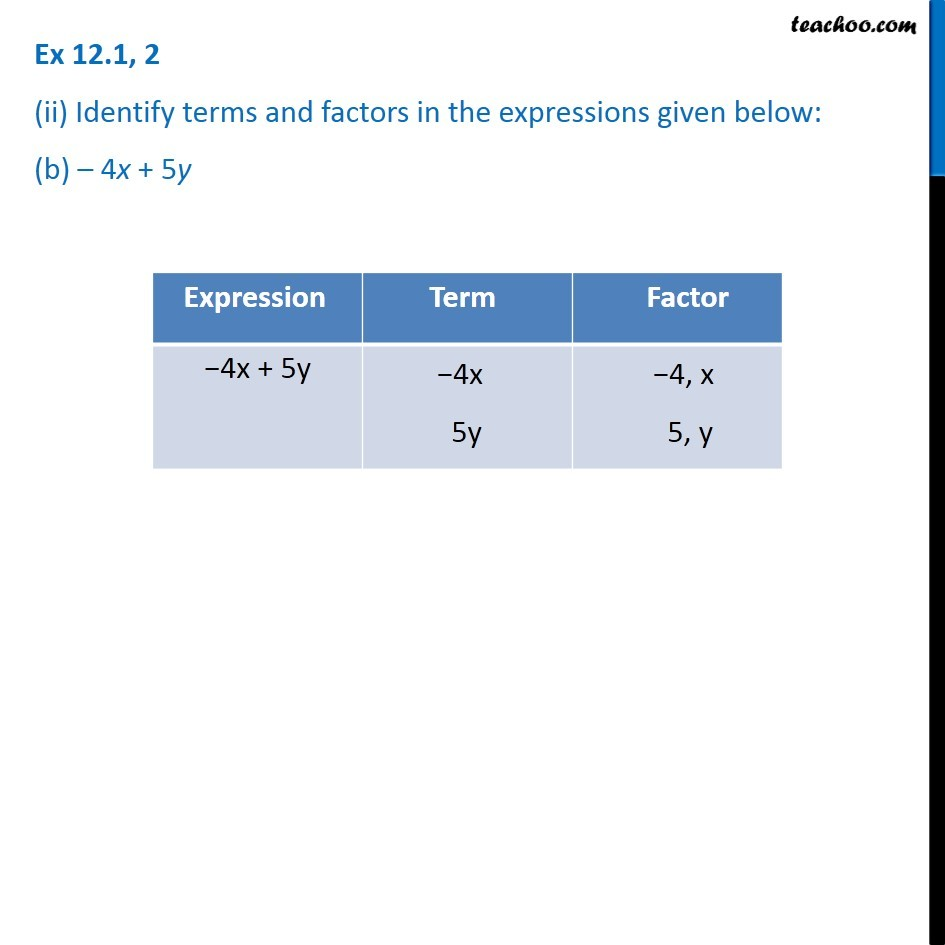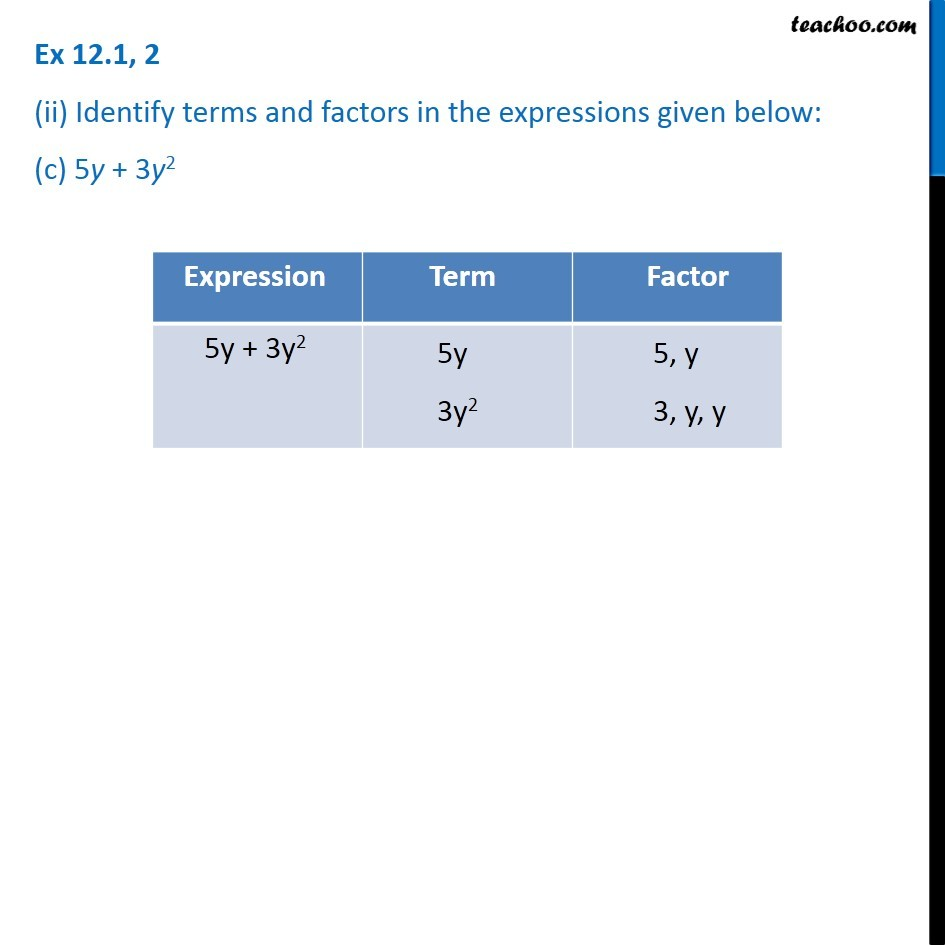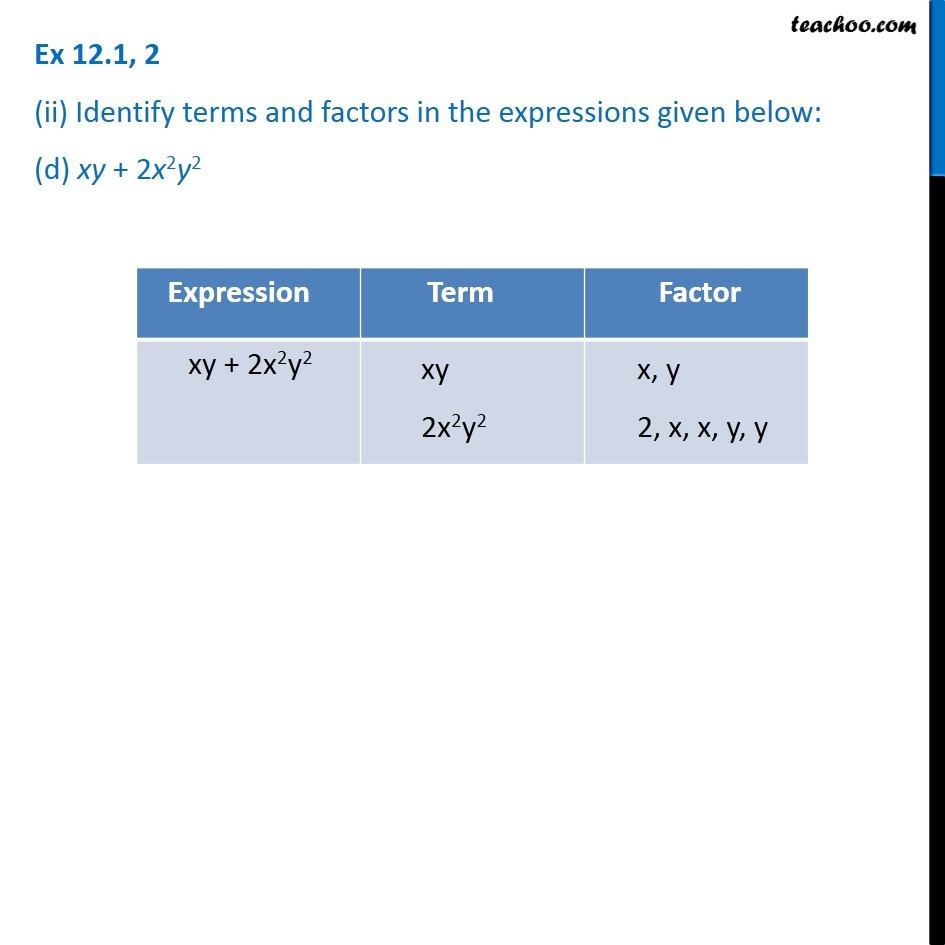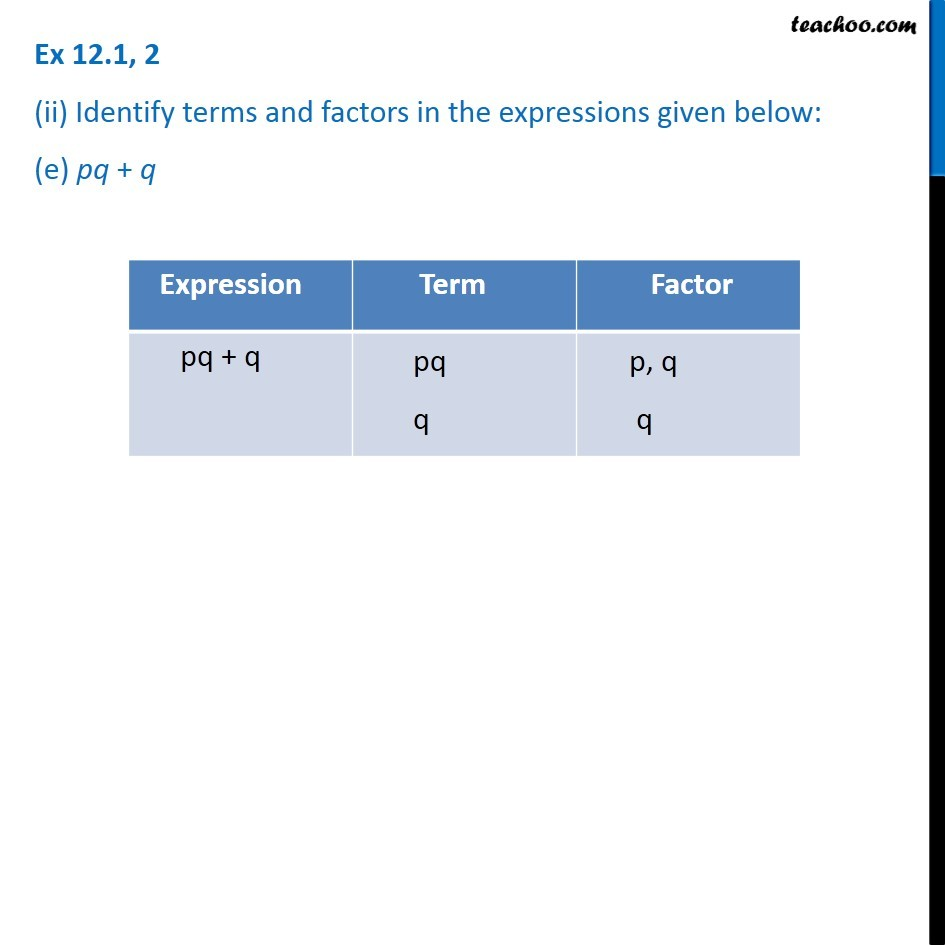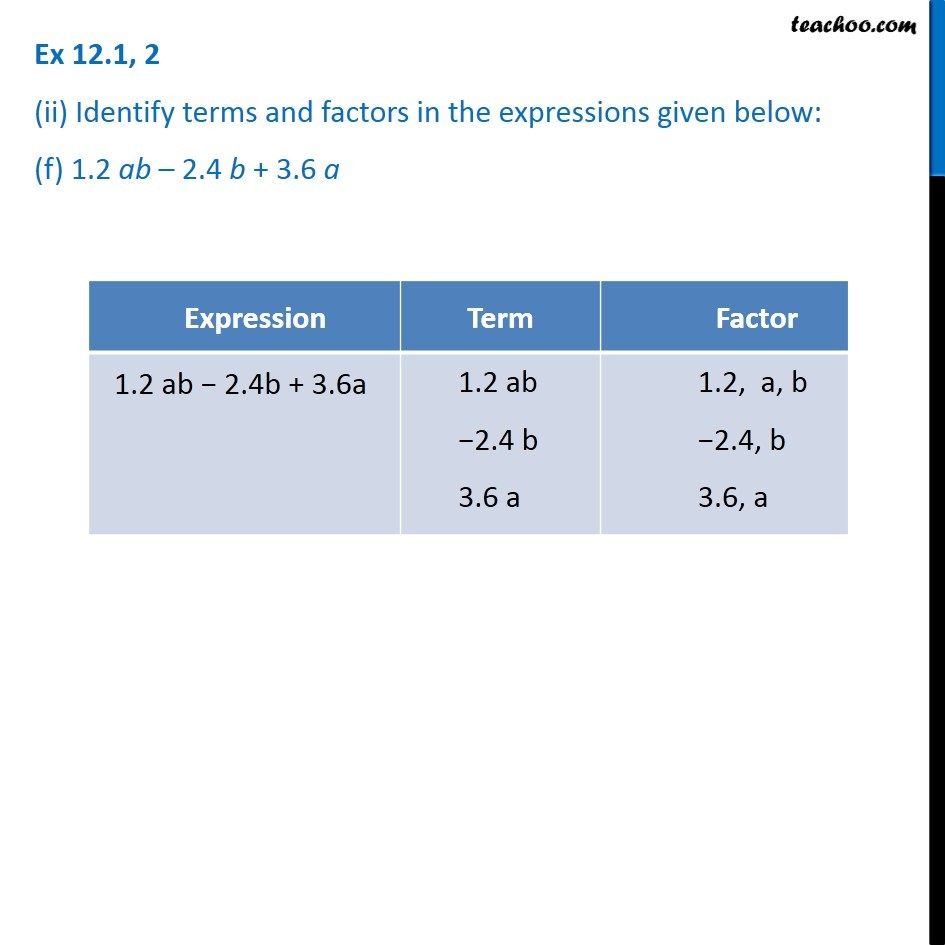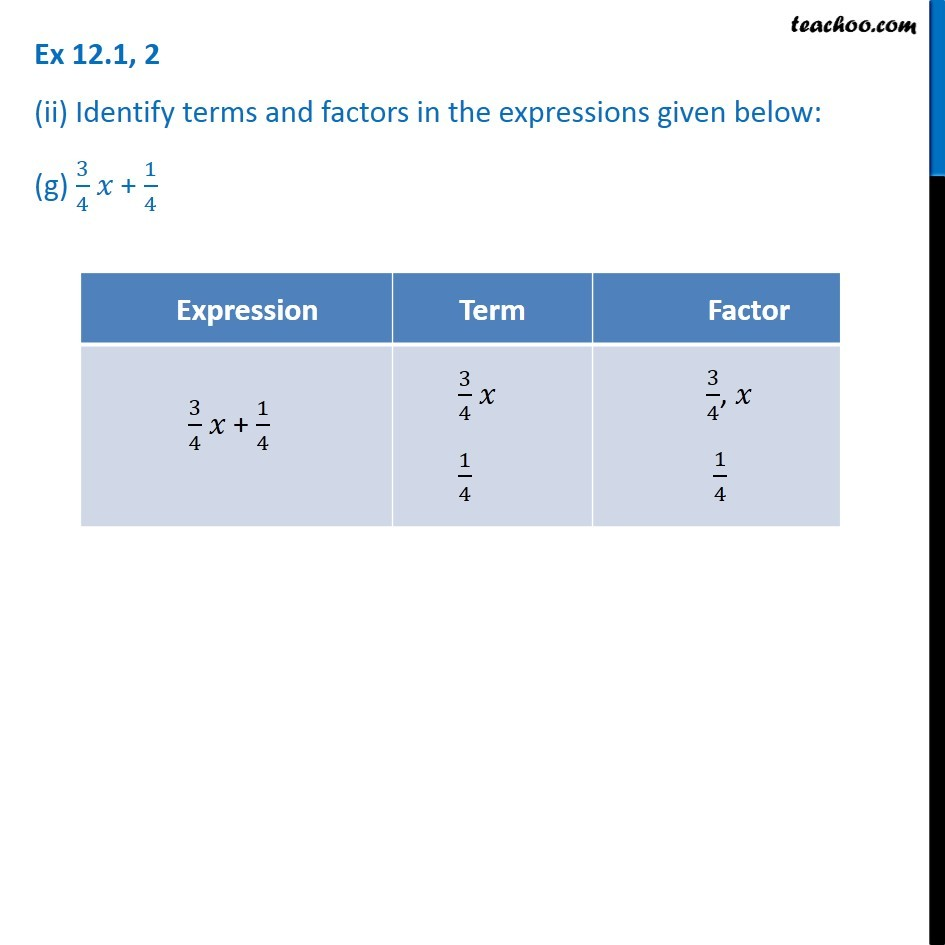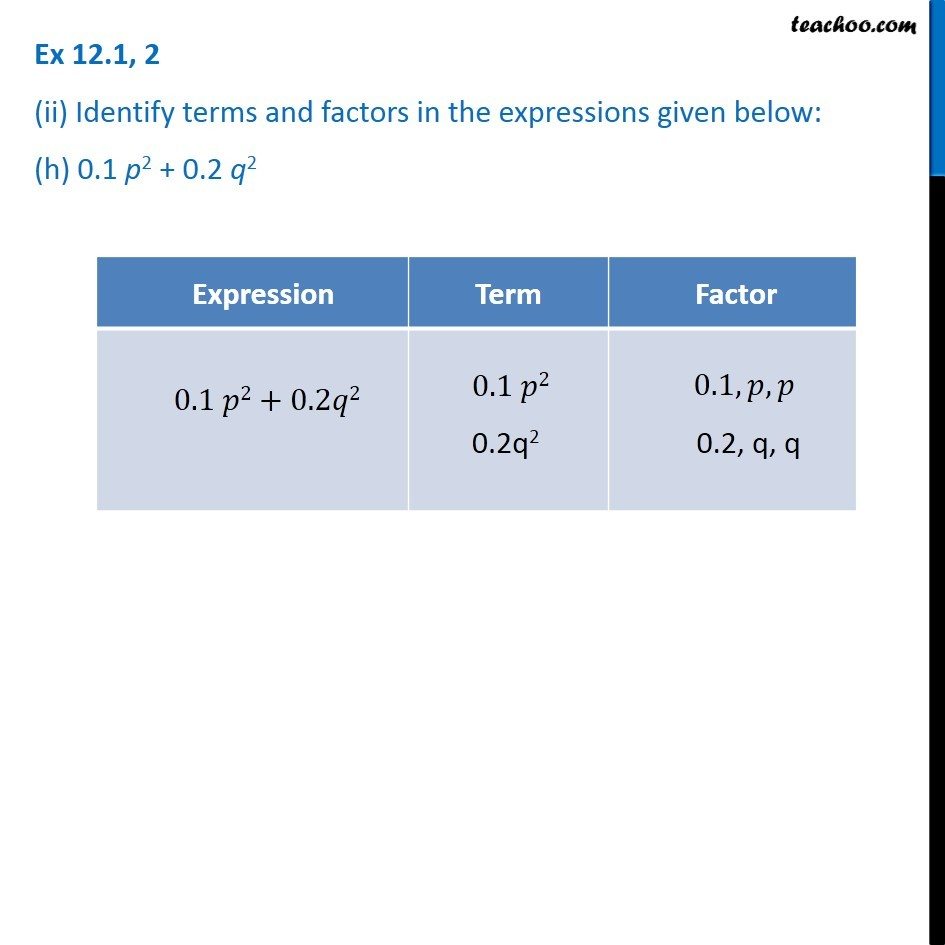1. Chapter 12 Class 7 Algebraic Expressions
2. Concept wise
3. Terms, Factors and Coefficients

Transcript

Ex 12.1, 2 (i) Identify the terms and their factors in the following expressions Show the terms and factors by tree diagrams. (a) x – 3 Ex 12.1, 2 (i) Identify the terms and their factors in the following expressions Show the terms and factors by tree diagrams. (b) 1 + x + x2 Ex 12.1, 2 (i) Identify the terms and their factors in the following expressions Show the terms and factors by tree diagrams. (c) y – y3 Ex 12.1, 2 (i) Identify the terms and their factors in the following expressions Show the terms and factors by tree diagrams. (d) 5xy2 + 7x2y 5xy2 + 7x2y 5xy2 Ex 12.1, 2 (i) Identify the terms and their factors in the following expressions Show the terms and factors by tree diagrams. (d) 5xy2 + 7x2y Ex 12.1, 2 (i) Identify the terms and their factors in the following expressions Show the terms and factors by tree diagrams. (e) – ab + 2b2 – 3a2 −ab + 2b2 − 3a2 Ex 12.1, 2 (ii) Identify terms and factors in the expressions given below: (a) – 4x + 5 Ex 12.1, 2 (ii) Identify terms and factors in the expressions given below: (b) – 4x + 5y Ex 12.1, 2 (ii) Identify terms and factors in the expressions given below: (b) – 4x + 5y Ex 12.1, 2 (ii) Identify terms and factors in the expressions given below: (c) 5y + 3y2 Expression Term Factor -4x + 5 4x -4x 3 5 Ex 12.1, 2 (ii) Identify terms and factors in the expressions given below: (b) – 4x + 5y Ex 12.1, 2 (ii) Identify terms and factors in the expressions given below: (c) 5y + 3y2 Ex 12.1, 2 (ii) Identify terms and factors in the expressions given below: (e) pq + q Ex 12.1, 2 (ii) Identify terms and factors in the expressions given below: (f) 1.2 ab – 2.4 b + 3.6 a Ex 12.1, 2 (ii) Identify terms and factors in the expressions given below: (g) 3/4 𝑥 + 1/4

Terms, Factors and Coefficients